### Home > GB8I > Chapter 3 Unit 4 > Lesson INT1: 3.2.1 > Problem3-77

3-77.

For each of the polygons formed by algebra tiles below:

• Sketch and label the shape on your paper and write an expression that represents the perimeter.

• Simplify your perimeter expression as much as possible.

1.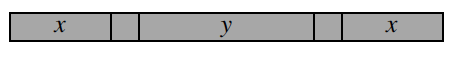• Start by labeling the sides.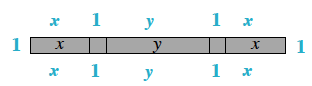$x+1+y+1+x+1+x+1+y+1+x+1$
Combine like terms.

$4x+2y+6$

1.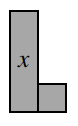• See the help for part (a).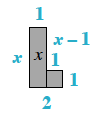$2x+4$

1.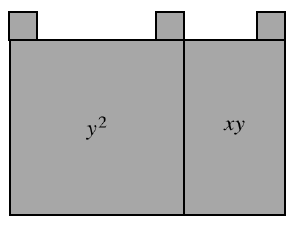•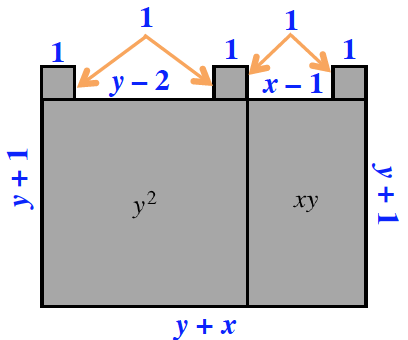$2x+4y+6$1.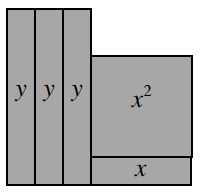• See the help for part (a).

$2x+2y+6$